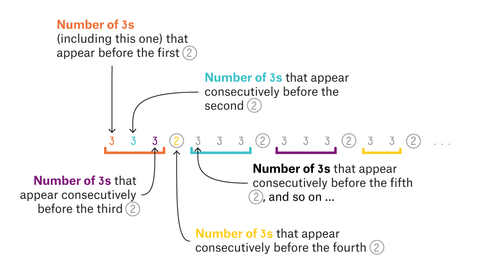Each week FiveThirtyEight posts a mathematical riddle to solve over the weekend. This latest week's problem was interesting, and I wanted to post my solution. The original problem is:

Take a look at this string of numbers:

333 2 333 2 333 2 33 2 333 2 333 2 333 2 33 2 333 2 333 2 …

At first it looks like someone fell asleep on a keyboard. But there’s an inner logic to the sequence:This sequence creates itself, number by number, as explained in the image above. Each digit refers to the number of consecutive 3s before a certain 2 appears. Specifically, the first digit refers to the number of consecutive 3s that appear before the first 2, the second digit refers to the number of 3s that appear consecutively before the second 2, and so on toward infinity.

The sequence never ends, but that won’t stop us from asking us questions about it. What is the ratio of 3s to 2s in the entire sequence?

Let's first implement the sequence in Python. We can use a queue to hold the sequence, and when we pop a new element off the queue, we can extend the queue (adding three 3s and a 2, or two 3s and a 2) based on the value of the popped element.

We could brute force the solution by computing the ratio as we extend the sequence ever longer, but this will only ever give us an approximation, and no realisation as to why the solution is so.

I'm going to show off how I played around with the problem, naively, and then started to formulate a solution. The first thing I computed was the ratio of 2s present in the sequence. This isn't the desired answer (ratio of 3s over 2s), but the ratio of 2s, which i'll call $$R$$, can be used to find the original solution too: $$\frac{1- R}{R}$$. Also, $$R$$ can be seen as a probability, something that didn't turn out to be useful but was a possible attack. Using the Python code, R was computed to be approximately 0.2679491. So I had some place to start.

Next I looked at where the 2s were placed in the sequence. Ex: position 4, 8, 12, 15, and so on were 2s. Extending, 2s were placed at positions:

4, 8, 12, 15, 19, 23, 27, 30, 34, 38, 42, 45, 49, 53, 56, 60, 64, 68, ...

Inspecting this, it's clear that 2s will occur every fourth number, except every 4th time, when it appears after three numbers. Call this last exception a "skip". Playing around with this pattern, I constructed a crude function to estimate the position of the $$n$$th 2, $$4n - 4n / (16)$$. (I didn't simplify this to make it more clear: the first term is where I expect the position to be given there were no "skips"; the second term is the estimated number of skips after $$4n$$ elements of the sequence)

It gave pretty close results for values of $$n$$ under 50. I could also estimate $$R$$ by looking at $$\frac{n}{4n - 4 n / 16}$$. For large $$n$$, this gave a number pretty close to my Python approximation of $$R$$ (above). But it wasn't close enough, and something was wrong. Simplifying the expression to $$\frac{1}{4 - \frac{1}{4}}$$ - I guessed that maybe this looks like a continued fraction! Trying a more accurate approximation $$\frac{1}{4 - \frac{1}{1 - \frac{1}{4}}}$$  gave an even better result! Newly motivated, I went back to my "crude approximation function". In the limit, the correct function would look like $$4n - Rn$$. Why? Again, given no "skips" we expect the $$n$$th 2 to be at $$4n$$, then subtract the number of "skips", which is exactly the number of 2s, given by $$Rn$$. Thus $$R = \frac{1}{ 4 - R}$$. (This confirms the continued fraction). Solving the quadratic, $$R = 2 - \sqrt{3} \approx 0.26794919$$.

Now we can solve the original problem, given by $$\frac{1- R}{R} = \frac{\sqrt{3} - 1}{2 - \sqrt{3}} \approx 2.7320508$$.

[Image credit to FiveThirtyEight]